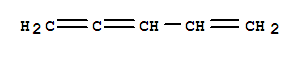# Question #e4dc9

Aug 25, 2016

Here are several possibilities.

#### Explanation:

The corresponding alkane would have the formula ${\text{C"_5"H}}_{12}$.

Each ring or double bond in the molecule removes 2 $\text{H}$ atoms.

Our formula is missing 6 $\text{H}$ atoms, so

$\frac{\text{Rings + double bonds" = "12 - 6}}{2} = \frac{6}{2} = 3$

There are six possible combinations.

I will give one example of each type.

3 Double bondsPenta-1,2,4-triene has 6 $\text{C-H}$ σ bonds, 4 $\text{C-C}$ σ bonds, and 3 $\text{C-C}$ π bonds (10 σ + 3 π).

1 Triple bond + 1 double bondPent-3-en-1-yne has 6 $\text{C-H}$ σ bonds, 4 $\text{C-C}$ σ bonds, and 3 $\text{C-C}$ π bonds (10 σ + 3 π).

1 Ring + 1 triple bondEthynylcyclopropane has 6 $\text{C-H}$ σ bonds, 5 $\text{C-C}$ σ bonds, and 2 $\text{C-C}$ π bonds (11 σ + 2 π).

1 Ring + 2 double bondsCyclopenta-1,3-diene has 6 $\text{C-H}$ σ bonds, 5 $\text{C-C}$ σ bonds, and 2 $\text{C-C}$ π bonds (11 σ + 2 π).

2 Rings + 1 double bondBicyclo[2.1.0]pent-2-ene has 6 $\text{C-H}$ σ bonds, 6 $\text{C-C}$ σ bonds, and 1 $\text{C-C}$ π bond (12 σ + 1 π).

Three ringsTricyclo[1.1.1.0]pentane has 6 $\text{C-H}$ σ bonds, 7 $\text{C-C}$ σ bonds, and 0 $\text{C-C}$ π bonds (13 σ + 0 π).